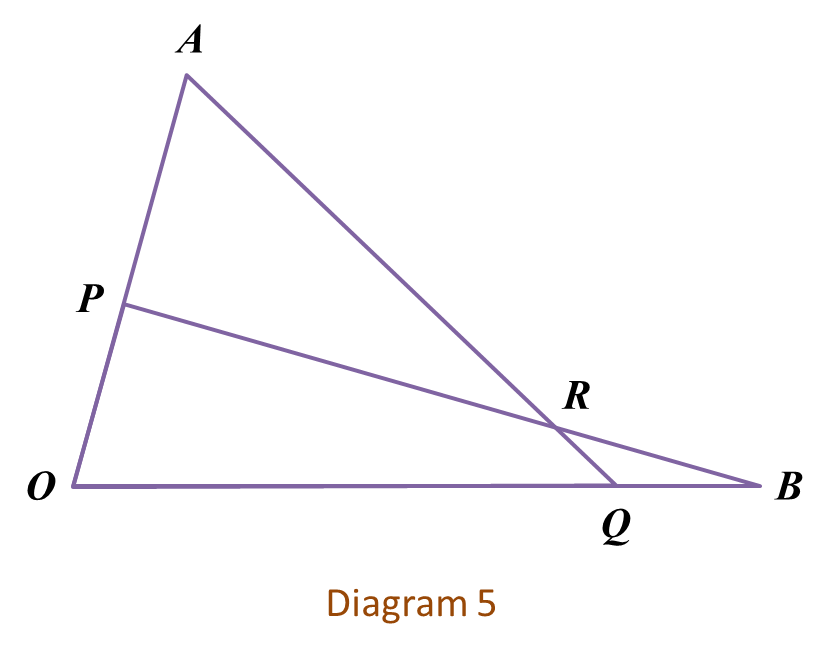\

# SPM Additional Mathematics 2018, Paper 2 (Question 7 – 9)

Question 7 (10 marks):

Solution:

$\begin{array}{l}\left(b\right)\left(i\right)\\ \mathrm{sin}\left(\frac{3x}{2}+\frac{\pi }{6}\right)-\mathrm{sin}\left(\frac{3x}{2}-\frac{\pi }{6}\right)=\frac{1}{2},0\le x\le 2\pi \\ \mathrm{cos}\frac{3x}{2}=\frac{1}{2}\\ \frac{3x}{2}=\frac{\pi }{3},\left(2\pi -\frac{\pi }{3}\right),\left(2\pi +\frac{\pi }{3}\right)\\ \frac{3x}{2}=\frac{\pi }{3},\frac{5\pi }{3},\frac{7\pi }{3}\\ x=\frac{2\pi }{9},\frac{10\pi }{9},\frac{14\pi }{9}\end{array}$Question 8 (10 marks):
Diagram 5 shows triangles OAQ and OPB where point P lies on OA and point Q lies on OB. The straight lines AQ and PB intersect at point R.Solution
(a)(i)

(a)(ii)

(b)

(c)

Question 9 (10 marks):
A study shows that the credit card balance of the customers is normally distributed as shown in Diagram 6.(a)(i) Find the standard deviation.
(ii) If 30 customers are chosen at random, find the number of customers who have a credit card balance between RM1800 and RM3000.
(b) It is found that 25% of the customers have a credit card balance less than RM y.
Find the value of y.

Solution:
(a)(i)
$\begin{array}{l}\mu =2870,x=3770\\ P\left(X>3770\right)=15.87%\\ P\left(Z>\frac{3770-2870}{\sigma }\right)=0.1587\\ P\left(Z>1.0\right)=0.1587\\ \frac{3770-2870}{\sigma }=1.0\\ \sigma =900\end{array}$

(a)(ii)

(b)
$\begin{array}{l}\mu =2870,x=y\\ P\left(x